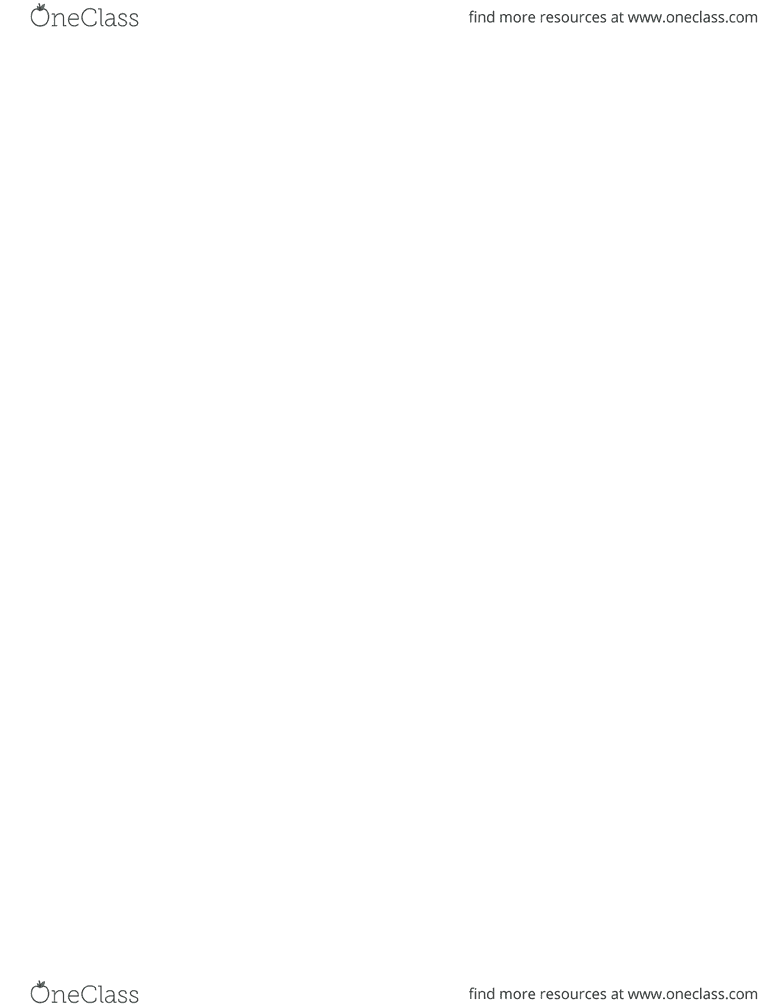Class Notes (1,100,000)
CA (620,000)
Western (60,000)
Kinesiology (4,000)
2241A/B (400)
Lecture 11

# Kinesiology 2241A/B Lecture Notes - Lecture 11: Centripetal Force, Angular Velocity, Bobsleigh

Department
Kinesiology
Course Code
Kinesiology 2241A/B
Professor
Daniel Bechard
Lecture
11

This preview shows page 1. to view the full 4 pages of the document.Angular Kinetics, Torques, Moments, Levers
Angular Kinetics
= Causes of Angular Movement
Kinematics:
How things move in a circle, how things rotate, what you see, position displacement velocity
when things move around a circle
Centripetal Force
Force causing centripetal acceleration, curvilinear path
Fc=M*W^2*r(kg m/s^2)
Draws an object to center of rotation.
Object wants to stay in certain path unless force acts upon it. Newton’s laws.
3 components:
- Mass of object
- Square of angular velocity
- Radius at which it works
If all three are larger it creates more centripetal force
Centrifugal Force
Center fleeing force
Is equal and opposite to centripetal
Has the same magnitude and dimensions but in opposite direction
Forces in Action
Bobsledding
- Forces act in ways that come in different shapes forms and sizes.
- Friction, try to minimize
- Gravity
- Wind resistance, fluid drag (amount of force created by wind)
- Centripetal, pulls toward an axis of rotation.
- Centrifugal, pulls away from an axis of rotation.
Torque Defined ( AKA Moment)
Force causing rotation
Causes a system/body to rotate
Torque is just an angular force
T= FxFA
Force measured in N
Force arm is measured in M, amount of distance between force application and pivot.
Torque measured in Nm
Big torque= increase in force generated, or increase in force arm
Properties of a Force
1) Magnitude
###### You're Reading a Preview

Unlock to view full version